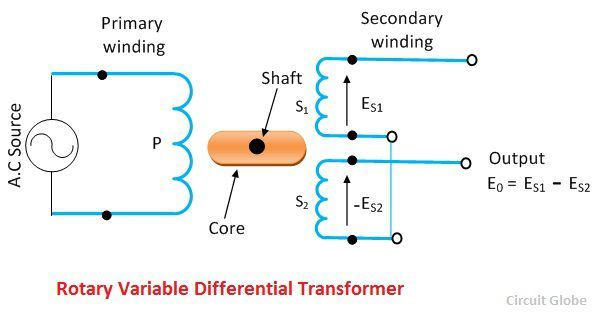# Rotary Variable Differential Transformer (RVDT)

Definition: The transformer which senses the angular displacement of the conductor is known as the Rotary Variable Differential Transformer or RVDT. It is the type of electromechanical transducer which gives the linear output proportional to the input angular displacement.

The circuit of RVDT is shown in the figure below. The working of the RVDT is similar to the LVDT. The only difference is that the LVDT uses the soft iron core for measuring the displacement, whereas the RVDT uses the cam shape core rotated between the primary and secondary winding with the help of the shaft.

### Theory of RVDT

The ES1 and the ES2 are the secondary voltage, and it varies with the angular displacement of the shaft.The G is the sensitivity of the RVDT. The secondary voltage is determined by the help of equation shown below.The difference between ES1 – ES2 gives a proportional voltage.The sum of the voltage is given by constant C.## Operation of LVDT

When the core is in the null position, the output voltages of the secondary winding S1 and S2 are equal and opposite. The net output of null position is zero. Any angular displacement from the null position will give the differential output voltage. The angular displacement is directly proportional to the differential output voltage. The response of the RVDT is linear.The differential output voltage of the transformer increases when the shaft rotates in a clockwise direction. And it decreases when the shaft moves in an anti-clockwise direction. The magnitude of the output voltage depends on the angular displacement and the direction of the shaft.